• python添加数组元素An array can be ... 可以通过在Python中使用“数组”模块来声明数组 。 Syntax to import "array" module: 导入“数组”模块的语法： import array as array_alias_name Here, impor...
python添加数组元素An array can be declared by using "array" module in Python.
可以通过在Python中使用“数组”模块来声明数组 。
Syntax to import "array" module:
导入“数组”模块的语法：
    import array as array_alias_name

Here, import is the command to import Module, "array" is the name of the module and "array_alias_name" is an alias to "array" that can be used in the program instead of module name "array".
在这里， import是导入Module的命令， “ array”是模块的名称， “ array_alias_name”是“ array”的别名，可以在程序中使用它而不是模块名称“ array” 。
Array declaration:
数组声明：
To declare an "array" in Python, we can follow following syntax:
要在Python中声明“数组” ，我们可以遵循以下语法：
    array_name   =   array_alias_name.array(type_code, elements)

Here,
这里，
array_name is the name of the array. array_name是数组的名称。 array_alias_name is the name of an alias - which we define importing the "array module". array_alias_name是别名的名称-我们定义了导入“ array module”的名称 。 type_code is the single character value – which defines the type of the array elements is the list of the elements of given type_code. type_code是单个字符值–定义数组元素的类型是给定type_code的元素列表。  添加元素 (Adding elements)
We can add elements to an array by using Array.insert() and Array.append() methods in Python.
我们可以使用Python中的Array.insert()和Array.append()方法将元素添加到数组中。
Example:
例：
# Adding Elements to an Array in Python

# importing "array" modules
import array as arr

# int array
arr1 = arr.array('i', [10, 20, 30])

print ("Array arr1 : ", end =" ")
for i in range (0, 3):
print (arr1[i], end =" ")
print()

# inserting elements using insert()
arr1.insert(1, 40)

print ("Array arr1 : ", end =" ")
for i in (arr1):
print (i, end =" ")
print()

# float array
arr2 = arr.array('d', [22.5, 33.2, 43.3])

print ("Array arr2 : ", end =" ")
for i in range (0, 3):
print (arr2[i], end =" ")
print()

# inserting elements using append()
arr2.append(54.4)

print ("Array arr2 : ", end =" ")
for i in (arr2):
print (i, end =" ")
print()

Output
输出量
Array arr1 :  10 20 30
Array arr1 :  10 40 20 30
Array arr2 :  22.5 33.2 43.3
Array arr2 :  22.5 33.2 43.3 54.4


翻译自: https://www.includehelp.com/python/adding-elements-to-an-array.aspxpython添加数组元素
展开全文列表 numpy 数据结构 数据分析
• 使用者都知道，python数组不仅是不需要声明长度，而且可以存储其它类型的元素 好，废话不多说，我们直接来看图片 看图片可知，数组的前两个一个存储的是数组存储的总长度，另一个存储的是当前元素的长度，每当...
python中数组的存储数据结构

python的数组和其它语言的数组底层有很大的不同
使用者都知道，python的数组不仅是不需要声明长度，而且可以存储其它类型的元素

好，废话不多说，我们直接来看图片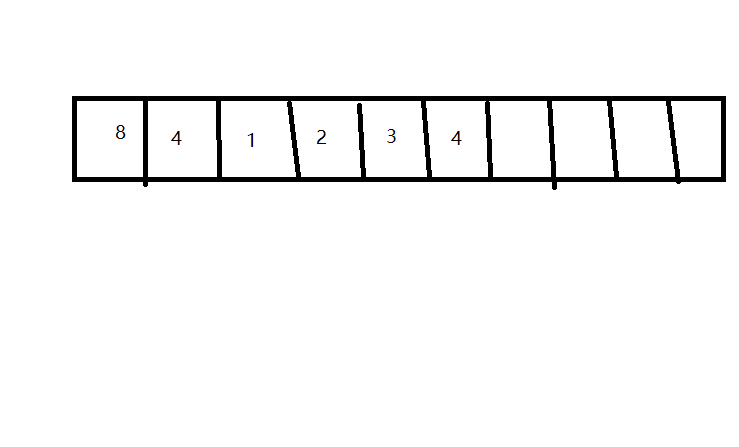看图片可知，数组的前两个一个存储的是数组存储的总长度，另一个存储的是当前元素的长度，每当数组多一个元素的时候，第二格的数据加1，直到总空间大于第一格的数字时，数组自动成倍扩展，即第一格变成16


展开全文• python数组声明如何避免浅拷贝 声明一个初始元素都为0的10*10的二维数组 # 错误的声明方法，会造成浅拷贝 a = [*10]*10 这样实际是一种拷贝，即a中拷贝了10个一样的[0,0,0,0,0,0…0] 一旦修改了例如a...
python 空数组的声明如何避免浅拷贝
声明一个初始元素都为0的10*10的二维数组
# 错误的声明方法，会造成浅拷贝
a = [*10]*10

这样实际是一种拷贝，即a中拷贝了10个一样的[0,0,0,0,0,0…0]
一旦修改了例如a的值，那么所有a[i]的值都变化了
# 正确的声明方法
a = [*10 for i in range(10)]



展开全文浅拷贝
• 当我们声明一个列表list，并向其中添加元素，一旦元素数量超过底层数组的大小，列表类会向系统重新申请一个更大的底层数组，并使这个更大的底层数组的前面部分和原来的数字一样。这时原来的数组就不需要了，会给系统...
< Data Structures andAlgorithms in Python > Michael T.Goodrich,Roberto Tamassia,Michael H.Goldwasser 学习笔记
python中创建数组，计算机系统先会创建一个低层次数组，以便系统为其存储分配连续内存，通常情况下，底层数组会比列表的长度更长。当我们声明一个列表list，并向其中添加元素，一旦元素数量超过底层数组的大小，列表类会向系统重新申请一个更大的底层数组，并使这个更大的底层数组的前面部分和原来的数字一样。这时原来的数组就不需要了，会给系统回收，这样一来，可以继续向列表中添加元素了（元组和字符串不需要考虑，因为元组和字符串对象实例化后就不可变，底层数组大小也就确定了）。如下代码及其结果给出证明：
import sys
data = []
n = 20
for k in range(n):
a = len(data)
b = sys.getsizeof(data)
print('Length:{0:3d};Size in bytes:{1:4d}'.format(a,b))
data.append(None)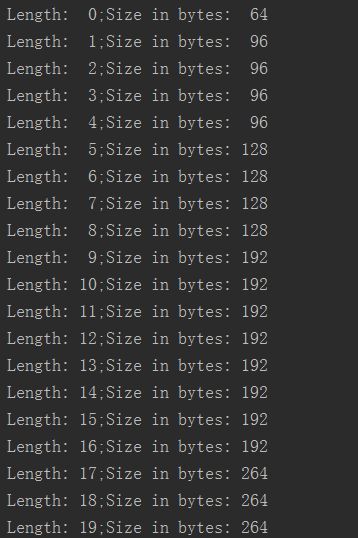sys模块中的getsizeof函数用于给出python中存储对象的字节数
在上面图片中可以看出，一个空列表占了64个字节的内存。python中每个列表对象除了存储元素之外，还需要存储一些状态，比如说列表当前存储的元素个数等。当我们添加第一个元素的时候，字节数从64调到了96，增加了32个字节，由于本实验是在64位机器上运行的，32个字节可以存储4个对象引用。这和我们之前所述一致，当对列表添加第2、3、4个元素的时候，占用的内存大小并没有变化，以此类推当我们添加第5、9、17个元素的时候，系统都会给列表重新分配新的底层数组，以便存储更多的元素。
实现动态数组
当底层数组$A$已满时，需要添加元素，系统会进行如下操作：

分配一个更大的数组$B$；
令$B[i]=A[i]（i=0，1，……，n-1）$；
令$A=B$，即之后使用$B$来代替$A$；
释放原数组所占用的内存。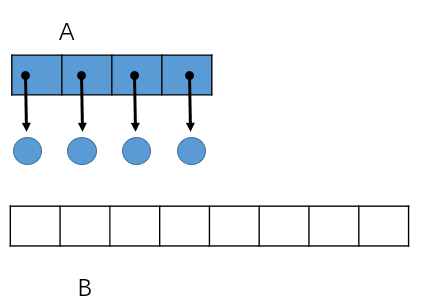创建数组$B$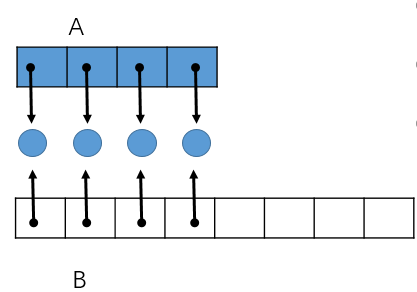把A中元素存入$B$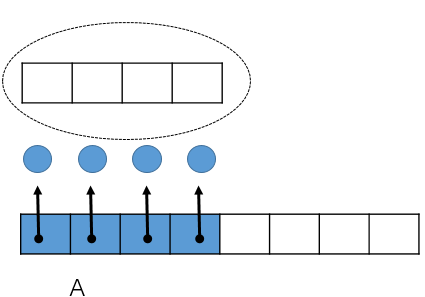释放内存

摊销分析
根据以上分析，data.append(None)操作的时间复杂度不是每次都是$O(1)$，当底层数组已满时，进行data.append(None)操作，其时间复杂度就会变为$O(n)$，n为原来底层数组的大小。所以列表append操作的时间复杂度和底层数组的大小有关系，如果底层数组大小无限大，那append操作的时间复杂度就是$O(1)$，但是这样会占过多的内存，明显不可取。每次底层数组存满，创建一个多大的底层数组来替换原先的底层数组会很大程度地影响append的性能。我们需要在运行效率和内存使用之间选取有个折中的方案。
大小按几何增长
假如当前底层数组大小为c，当底层数组存满时，系统创建新的底层数组，其大小为2c（c的倍数）。我们能够证明这样的机制摊销运行时间为$O(1)$，证明如下：
我们假设底层数组初始大小为c，每次增长原来底层数组的2倍。第一次添加c个元素，其时间复杂度为$O(c)$，再添加c个元素，其时间复杂度为$O(2c)$（底层数组满时，需要创建数组并将原来的数据存入新数组，时间复杂度为$O(c)$，再加上之后添加的c个元素，其时间复杂度为$O(2c)$），再添加2c个元素，其时间复杂度为$O(4c)$，再添加8c个元素，其时间复杂度为$O(16c)$，以此类推。
如图：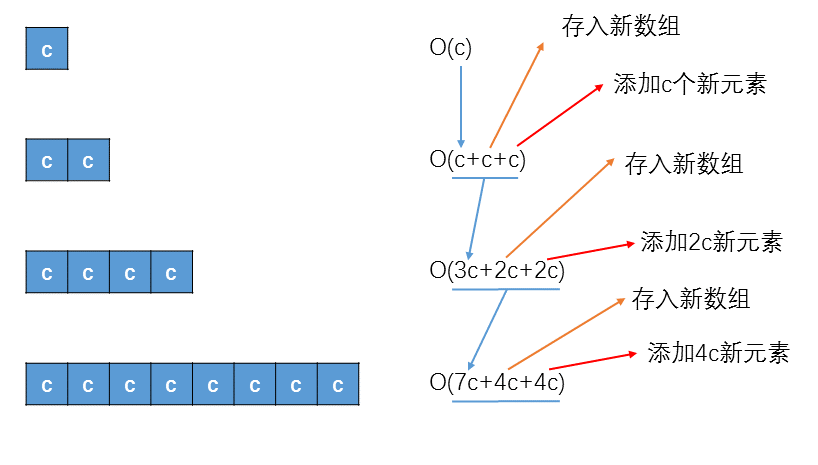添加元素
c
c
2c
4c
8c
……

添加时间复杂度
O(c)
O(2c)
O(4c)
O(8c)
O(16c)
……

总元素n
c
2c
4c
8c
16c
……

总时间复杂度
O(c)
O(3c)
O(7c)
O(15c)
O(31c)
……

$n=c+\frac{c(1-2^m)}{1-2}=2^mc$
$总时间复杂度=O\left(\frac{c(1-2^m)}{1-2}\right)=O\left((2^{m+1}-1)c\right)$
根据上面的两个公式，我们可以得出时间复杂度为$O(2n-c)$
因此，每个添加操作的摊销运行时间为$O(1)$
避免使用固定增量
设固定增量为c，和上面分析的一样：设起始为空底层数组，大小为c，每添加c个元素所花费的时间为c，2c，3c，……，mc，总共添加了$n=mc$个元素。其总共花费的时间为
$\sum_{i=1}^mci=c*\sum_{i=1}^mi=c\frac{m(m+1)}{2}=\frac{n^2+c}{2c}$
因此，执行$n$个append操作花费的时间为$\Omega(n^2)$，这个的性能明显几何增长的要差。
Python列表的效率
data、data1、data2的长度分别为$n$、$n_1$、$n_2$

操作
运行时间

data[j] = val
$O(1)$

data.append(value)
$O(1)^*$

data.insert(k,value)
$O(n-k+1)^*$

data.pop()
$O(1)^*$

data.pop(k)del data[k]
$O(n-k)^*$

data.remove(value)
$O(n)^*$

data1.extend(data2)data1 += data2
$O(n_2)^*$

data.reverse()
$O(n)$

*    摊销 ：改变数组大小的操作，有些情况下需要改变底层数组，所以需要进行摊销的操作。


展开全文数据结构
• 本文转载自：https://www.cnblogs.com/alibai/p/4027324.html 作者：alibai 转载请注明该声明。 增加时a.append( 'a ')就可以了。只要按顺序加，就没有问题 。 使用时，完全可以使用下标： 代码如下 复制代码 a[0...介绍
• python 三维数组声明 python 动态数组声明
• 版权声明：下面链接为原博主原创文章，遵循 CC 4.0 BY-SA 版权协议，转载请附上原文出处链接和本声明。 转载链接：https://blog.csdn.net/fu6543210/article/details/83240024 ...
• Python 没有数组概念 ，使用列表（list）来实现的，罗列几个基本操作： 声明 一维 demo = [] 动态大小数组，成员数可变 demo =，静态大小数组，三个成员，标号从0开始 demo = [“a”,“b”] 数组初值 二维 demo =...
• 如何在Python声明数组？ 我在文档中找不到任何对数组的引用。arrays
• Declaration/creation of the Array数组声明/创建 Common Data Items and Methods of an Array in PythonPython数组的通用数据项和方法 Here are the examples to demonstrate how to access elements from t...numpy 动态规划 perl
• 列表是 Python数组的一种表现形式，列表没有固定大小。 但是有时候需要一个固定大小的列表，并且需要事先指定每个元素初始值都为0。 可以如下声明： L = *n 上面定义了大小为n，初始值全为0的列表 ...
• 那么以此类推，是不是[ * n] * n就可以声明二维数组了呢。 答案是否定的。 因为 [] * n和 Java的clone() 函数是一样的，属于"浅拷贝"。 也就是说，[ * n] * n 是把  * n的“指针” 拷贝了n份。也就是...
• 本文转载自：...其他语言中遍历数组: for (int i = 0; i < list.Length; i++) { 　System.out.println(list[i]); } 　python中遍历数组: ...遍历 简单
• 实验的前提条件本文会出现一些编程实验，有兴趣实践的...语境中的Python数组数组是Python 语言原生支持的数据结构，直接用方括号声明即可，如：arr = [1,2,3,4,5]为了方便数组的创建，Python 支持递推表达式等优雅的写rgb
• 知识点：Numpy、数组 ...很多时候可以声明 axis。axis=0，表示沿着第 0 轴进行操作，即对每一列进行操作；axis=1，表示沿着第1轴进行操作，即对每一行进行操作。 NumPy 的数组中比较重要 ndarray 对象属性有： 1. nd.
• 声明：本人的所有博客皆为个人笔记，作为个人知识索引使用，因此在叙述上存在逻辑不通顺、跨度大等问题，希望理解。分享出来仅供大家学习翻阅，若有错误希望指出，感谢！ Python数字(Number) Python 数字数据类型...
• v = [[] for i in range(N)]
• #声明三维数组 num=[[[33,45,67],[23,71,66],[55,38,66]], \ [[21,9,15],[38,69,18],[90,101,89]]] value=num#设置main为num数组的第一个元素 for i in range(2): for j in range(3): for k in .....
• 版权声明：本文为CSDN博主「一骑走烟尘」的原创文章，遵循 CC 4.0 BY-SA 版权协议，转载请附上原文出处链接及本声明。 原文链接：https://blog.csdn.net/zgcr654321/article/details/88015849 如果对象...
• 文章目录题目:暴力求解：三次反转实现：使用数组切片的方法实现: ...接着声明一个变量来存储数组最后一个数，然后依次把数组后移，最后把变量的值赋值给数组的第一个元素。重复上面步骤k次。当k&g...算法 leetcode
• python里面数组可以声明一个未知长度的数值 比如python可以这样定义： #!/usr/bin/python3 a1=[] for i in range(0,10): a1[i]=str(i)+"a" #print(i) 执行报错：IndexError: list assignment index out of ...
• 函数参数声明 numpy.delete(arr, obj, axis=None) arr：输入数组 obj：切片，整数，表示哪个子数组要被移除 axis：删除子数组的轴 axis = 0：表示删除数组的行 axis = 1：表示删除数组的列 axis = None：表示把数组...
• 1 声明列表 names =["tome","jonson","black"] print(names) 2 访问列表元素 names = ["tomy","jonson","black"] print(names) #访问列表最后一个元素,使用-1下标 names = ["tomy","jonson",...
• c/c++ c# java 一维数组 datatype arrayname[size]; //声明并创建数组，不会初始... //声明数组的同时为元素赋值。如果在声明时为数组指定了数组元素，则不需要指定数组长度，编译器会自动...
• 本文转载自：https://www.cnblogs.com/huangshiyu13/p/6672828.html 作者：huangshiyu13 转载请注明该声明。...============改变数组的维度==================已知reshape函数可以有一维数组形成多维数组ra...连接 组合 numpy
• I'm trying to add items to an array in python. 我试图将项目添加到python中的数组。 I run 我跑 array = {} Then,arrays
• 最近在学python的数据分析...声明：作者用的是python2.7转置是重塑的一种特殊形式，他返回的是源数据的视图（不会进行任何复制操作，这一点要和花式索引不太一样，后者总是将数据复制到新数组中）。 完成转置可以通过numpy...

# python声明数组python 订阅